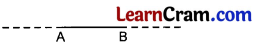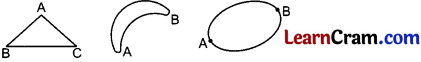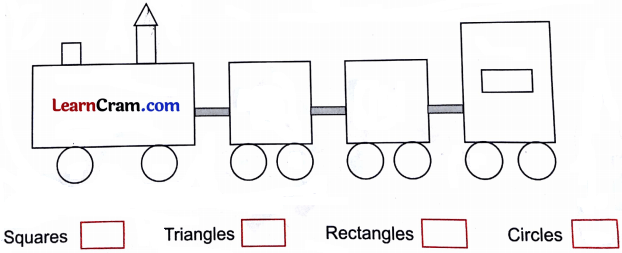# DAV Class 3 Maths Chapter 12 Worksheet 1 Solutions

The DAV Maths Book Class 3 Solutions and DAV Class 3 Maths Chapter 12 Worksheet 1 Solutions of Geometry offer comprehensive answers to textbook questions.

## DAV Class 3 Maths Ch 12 WS 1 Solutions

Question 1.
Name four objects having a plane edges.
Solution:
(i) Slate
(ii) Note (money)
(iii) Stamp
(iv) Book

Question 2.
Name four objects having a curved surface.
Solution:
(i) Football
(ii) Guave, apple, etc
(iii) Globe
(iv) Sun, moon, etc

Question 3.
Name four objects having both plane and curved surfaces.
Solution:
(i) Milk bottle
(ii) Pencil
(iii) Chalk
(iv) StickQuestion 4.

(a) Globe has a (plane/curved) surface.
Solution:
Curved surface

(b) Mirror has a (plane/curved) surface.
Solution:
Plane surface

(c) Playing card has a (plane/curved) surface.
Solution:
Plane surface

(d) Laddoo has a (plane/curved) surface.
Solution:
Curved surface

(e) Burfi has a (plane/curved) surface.
Solution:
Plane surface

(f) 100 rupee note has a (plane/curved) surface.
Solution:
Plane surface### DAV Class 3 Maths Chapter 12 Worksheet 1 Notes

• Part of a solid which we can see and touch is called the surface of the solid.
• Some common shapes of solids are cuboid, cube, cylinder, cone, sphere, etc.
• A dot is called a point which gives us idea of a location.
• A line segment has two end points which can be measured.
• A ray is a straight path which extends endlessly in one direction.
• We can measure a line segment with a scale.

Lines and Shapes

The part of a straight line between two points is called a line segment.
So between A and B we have line segment AB.One straight line can pass through two points.

It we draw curved line between two point A and B to continue the line by moving the pencil from B to A along a different path we will get a closed shape.

E.g.Flat ShapesPlane Surfaces and Curved Surfaces

The part of a solid which we can see and touch is called surface of the solid.Let us have fun with shapes

Question 1.
Today is Neha’s birthday. She is planning to give return gifts to all her friends. See the gifts packs given below and identify the shapes. [Textbook Page 129]Solution:
Conical, Square, Circle, Rectangle, Cylindrical.Question 2.
Count the number of squares, circles, rectangles and triangles from the picture given below. [Textbook Page 129]Solution:
Squares – 3
Triangle – 1
Rectangles – 7
Circles – 8.